# Affine scheme

(diff) ← Older revision | Latest revision (diff) | Newer revision → (diff)

A generalization of the concept of an affine variety, which plays the role of a local object in the theory of schemes. Letbe a commutative ring with a unit. An affine scheme consists of a topological spaceand a sheaf of rings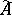on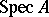. Here,is the set of all prime ideals of(called the points of the affine scheme), provided with the Zariski topology (or, which is the same, with the spectral topology), in which the basis of open sets is constituted by the subsets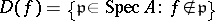, whereruns through the elements of. The sheaf of local ringsis defined by the condition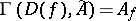, where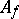is the localization of the ringwith respect to the multiplicative system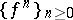, cf. Localization in a commutative algebra.

Affine schemes were first introduced by A. Grothendieck , who created the theory of schemes. A scheme is a ringed space which is locally isomorphic to an affine scheme.

An affine schemeis called Noetherian (integral, reduced, normal, or regular, respectively) if the ringis Noetherian (integral, without nilpotents, integrally closed, or regular, respectively). An affine scheme is called connected (irreducible, discrete, or quasi-compact, respectively) if the topological spacealso has these properties. The spaceof an affine scheme is always compact (and usually not Hausdorff).

The affine schemes form a category if the morphisms of these schemes, considered as locally ringed spaces, are considered as morphisms of affine schemes. Each homomorphism of ringsdefines a morphism of affine schemes: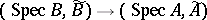, consisting of the continuous mapping(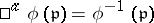for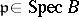), and a homomorphism of sheaves of rings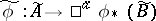, which transforms the section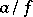of the sheafover the set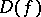into the section. The morphisms of an arbitrary scheme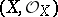into an affine scheme (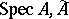) (which are also called-valued points of) are in a one-to-one correspondence with the homomorphisms of rings; thus, the correspondenceis a contravariant functor from the category of commutative rings with a unit into the category of affine schemes, which establishes an anti-equivalence of these categories. In particular, in the category of affine schemes there are finite direct sums and fibre products, dual to the constructions of the direct sum and the tensor product of rings. The morphisms of affine schemes which correspond to surjective homomorphisms of rings are called closed imbeddings of affine schemes.

The most important examples of affine schemes are affine varieties; other examples are affine group schemes (cf. Group scheme).

In a manner similar to the construction of the sheafit is possible to construct, for any-module, a sheaf of-modules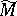onfor which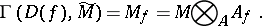Such sheaves are called quasi-coherent. The category of-modules is equivalent to the category of quasi-coherent sheaves of-modules on; projective modules correspond to locally free sheaves. The cohomology spaces of quasi-coherent sheaves over an affine scheme are described by Serre's theorem: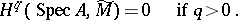The converse of this theorem (Serre's criterion for affinity) states that ifis a compact separable scheme, and if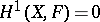for any quasi-coherent sheaf of-modules, thenis an affine scheme. Other criteria for affinity also exist , .

How to Cite This Entry:
Affine scheme. Encyclopedia of Mathematics. URL: http://encyclopediaofmath.org/index.php?title=Affine_scheme&oldid=15083
This article was adapted from an original article by V.I. DanilovI.V. Dolgachev (originator), which appeared in Encyclopedia of Mathematics - ISBN 1402006098. See original article